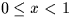Next: SLA_RANGE - Put Angle into Range
Up: SUBPROGRAM SPECIFICATIONS
Previous: SLA_PXY - Apply Linear Model

## SLA_RANDOM - Random Number

ACTION:
Generate pseudo-random real number in the range.
CALL:
R = sla_RANDOM (SEED)

GIVEN:

 R SEED an arbitrary real number

RETURNED:

 R SEED a new arbitrary value sla_RANDOM R Pseudo-random real number.

NOTE:
The implementation is machine-dependent.Next: SLA_RANGE - Put Angle into Range
Up: SUBPROGRAM SPECIFICATIONS
Previous: SLA_PXY - Apply Linear Model

SLALIB --- Positional Astronomy Library